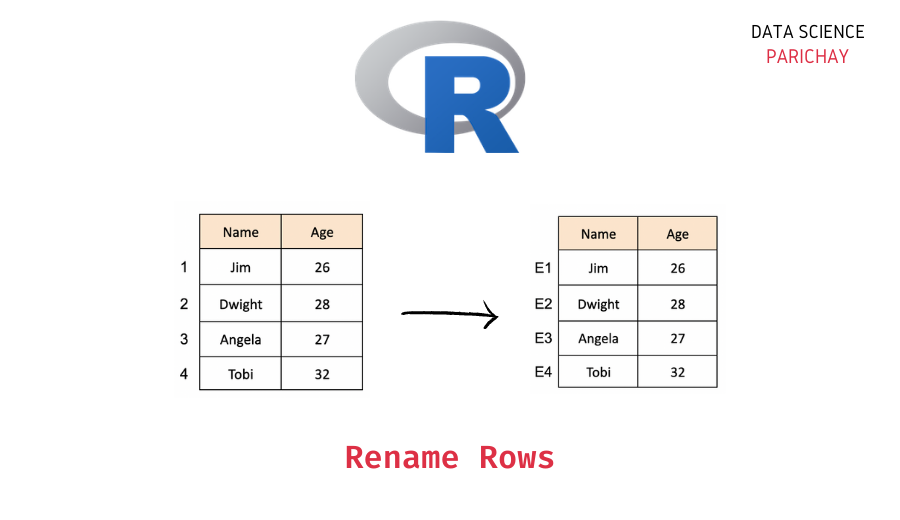# Rename Rows in R Dataframe (With Examples)

In this tutorial, we will look at how to rename the rows (also called the row indices) of a dataframe in R with the help of some examples.

## How to change the row names in an R dataframe?

You can use the built-in `rownames()` to rename the rows of a dataframe in R. It modifies the dataframe in place.

The following is the syntax to set the row names of a dataframe in R.

`rownames(dataframe) < c(r1, r2, r3, ...)`

Here, we use a vector containing the new row names to rename our dataframe’s rows.

Let’s now look at the usage of the above syntax with the help of a step-by-step example.

### Step 1 – Create a Dataframe

First, we will create a dataframe that we will be using throughout this tutorial.

```# create a dataframe
employees_df = data.frame(
"EmpID"= c("E1", "E2", "E3", "E4"),
"Age"= c(26, 28, 29, 32),
"Department"= c("Sales", "Sales", "Accounting", "HR")
)
# display the dataframe
print(employees_df)```

Output:

```  EmpID Age Department
1    E1  26      Sales
2    E2  28      Sales
3    E3  29 Accounting
4    E4  32         HR```

We now have a dataframe containing information about some employees in an office. The dataframe has the columns – “EmpID”, “Age”, and “Department”.

📚 Data Science Programs By Skill Level

Introductory

Intermediate ⭐⭐⭐

🔎 Find Data Science Programs 👨‍💻 111,889 already enrolled

Disclaimer: Data Science Parichay is reader supported. When you purchase a course through a link on this site, we may earn a small commission at no additional cost to you. Earned commissions help support this website and its team of writers.

Note that the row indices (or the row names) in the above dataframe are numbers starting from 1.

### Step 2 – Rename rows in the dataframe with `rownames()`

Now, let’s change the row names in the above dataframe to the respective employee names. For example, row 1 represents the employee with “EmplD” “E1” whose name is “Jim”.

Here, we want to rename the row from 1 to “Jim” and similarly for the other employees. For the sake of simplicity, we take an example where each employee has a different name.

Let’s rename the rows using a vector of employee name values and the `rownames()` function.

```# rename dataframe rows
rownames(employees_df) <- c("Jim", "Dwight", "Angela", "Tobi")
# display the dataframe
print(employees_df)```

Output:

```       EmpID Age Department
Jim       E1  26      Sales
Dwight    E2  28      Sales
Angela    E3  29 Accounting
Tobi      E4  32         HR```

You can see that the dataframe’s rows are renamed to the employee names. Notice that here we modified the dataframe in place.

Note that the new row names must be unique. That is, no two rows can have the same name. If you try to set the same name for multiple rows, you’ll get an error.

```# rename dataframe rows
rownames(employees_df) <- c("Jim", "Jim", "Angela", "Tobi")
# display the dataframe
print(employees_df)```

Output:

```Warning message:
“non-unique value when setting 'row.names': ‘Jim’”
Error in `.rowNamesDF<-`(x, value = value): duplicate 'row.names' are not allowed
Traceback:```

Here we get an error with the following message – “Error in `.rowNamesDF<-`(x, value = value): duplicate ‘row.names’ are not allowed“. This happened because we used the same row name, “Jim” for multiple rows.

## Summary – Rename Rows in R Dataframe

In this tutorial, we looked at how to change the row names of a dataframe in R. The following is a short summary of the steps mentioned in this tutorial.

1. Create a dataframe (skip this step if you already have a dataframe to operate on).
2. Rename the dataframe rows using the `rownames()` function. Here we set the row names of a dataframe to a vector of new row names.

You might also be interested in –

•